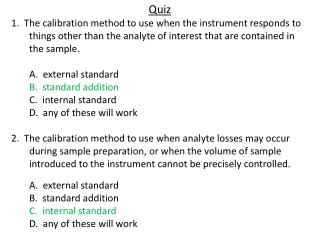# Quiz - PowerPoint PPT PresentationDownload PresentationQuiz

QuizDownload Presentation## Quiz

- - - - - - - - - - - - - - - - - - - - - - - - - - - E N D - - - - - - - - - - - - - - - - - - - - - - - - - - -
##### Presentation Transcript

1. Quiz 1. The calibration method to use when the instrument responds to things other than the analyte of interest that are contained in the sample. A. external standard B. standard addition C. internal standard D. any of these will work 2. The calibration method to use when analyte losses may occur during sample preparation, or when the volume of sample introduced to the instrument cannot be precisely controlled. A. external standard B. standard addition C. internal standard D. any of these will work

2. Quiz 3. Given the following linear regression for an external calibration, what is the concentration of a sample that gives an instrument response of 9362 and an instrument response of 650 for the blank? Show your work. y (response units) = 377(response units/ppm)x + 14.0 (response units) A. 24.8 ppm C. 9362 ppm B. 23.1 ppm D. none of the these 4. Given the equation of a line: y = 9.0x + 34, what is the x intercept? Show your work. A. 34 B. -34 C. -3.8 D. none of the above